# Fibonacci Numbers

Student
30 May 2013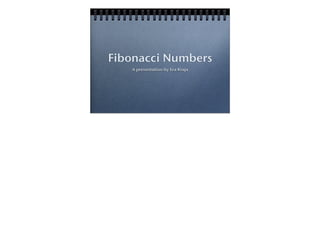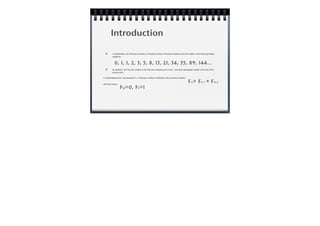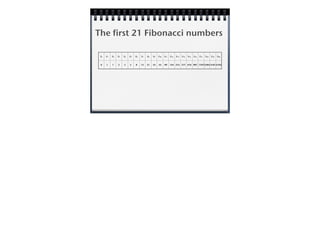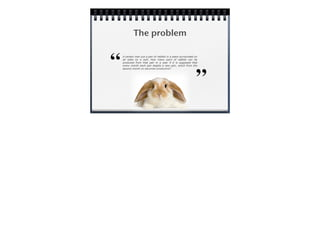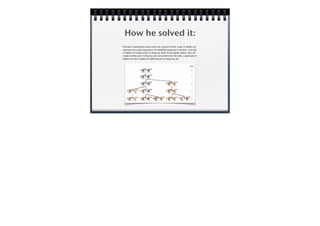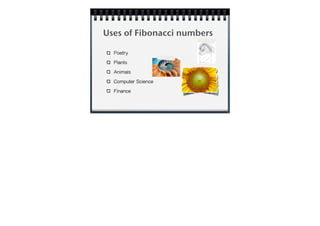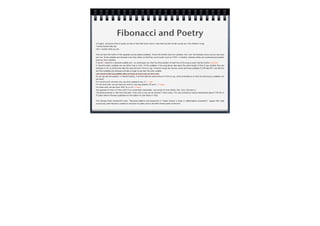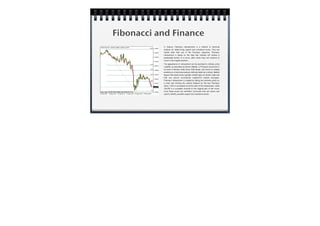1 sur 13

### Fibonacci Numbers

• 2. Who was Fibonacci? Also referred to as Leonard of Pisa, Fibonacci was an Itallian number theorist. It is believed that Leonardo Pisano Fibonacci was born in the 13th century, in 1170 (approximately) and that he died in 1250. Fibonacci was born in Italy but obtained his education in North Africa. Fibonacci is considered to be one of the most talented mathematicians for the Middle Ages. Few people realize that it was Fibonacci that gave us our decimal number system (Hindu-Arabic numbering system) which replaced the Roman Numeral system. When he was studying mathematics, he used the Hindu-Arabic (0-9) symbols instead of Roman symbols which didn't have 0's and lacked place value. In fact, when using the Roman Numeral system, an abacus was usually required. There is no doubt that Fibonacci saw the superiority of using Hindu-Arabic system over the Roman Numerals. He shows how to use our current numbering system in his book Liber abaci.
• 3. Introduction In mathematics, the Fibonacci numbers or Fibonacci series or Fibonacci sequence are the numbers in the following integer sequence: By definition, the first two numbers in the Fibonacci sequence are 0 and 1, and each subsequent number is the sum of the previous two. In mathematical terms, the sequence Fn of Fibonacci numbers is defined by the recurrence relation with seed values 0, 1, 1, 2, 3, 5, 8, 13, 21, 34, 55, 89, 144... Fn= Fn-1 + Fn-2 F0=0, F1=1
• 4. The first 21 Fibonacci numbers Fo F1 F2 F3 F4 F5 F6 F7 F8 F9 F10 F11 F12 F13 F14 F15 F16 F17 F18 F19 F20 0 1 1 2 3 5 8 13 21 34 55 89 144 233 377 610 987 15972584 4181 6765
• 5. The problem A certain man put a pair of rabbits in a place surrounded on all sides by a wall. How many pairs of rabbits can be produced from that pair in a year if it is supposed that every month each pair begets a new pair, which from the second month on becomes productive?
• 6. How he solved it: Fibonacci's experiment shows that over a period of time, a pair of rabbits will reproduce at a rate expressed in his identified sequence of numbers. One pair of rabbits will create a pair of offspring. When those rabbits mature, they will create another pair of offspring, and during that time, the older, original pair of rabbits will have created an additional pair of offspring, etc.
• 7. Uses of Fibonacci numbers Poetry Plants Animals Computer Science Finance
• 8. Fibonacci and Poetry In English, we tend to think of poetry as lines of text that rhyme, that is, lines that end with similar sounds as in this children's song: Twinkle twinkle little star How I wonder what you are. Also we have the rhythm of the separate sounds (called syllables). Words like twinkle have two syllables: twin- and -kle whereas words such as star have just one. Some syllables are stressed more than others so that they sound louder (such as TWIN- in twinkle), whereas others are unstressed and quieter (such as -kle in twinkle). If we let S stand for a stressed syllable and s an unstressed one, then the stress-pattern of each line of the song or poem has the rhythm SsSsSsS. In Sanskrit poetry syllables are are either long or short. All the syllables in the song above take about the same length of time to say whether they are stressed or not, so all the lines take the same amount of time to say. However cloudy sky has two words and three syllables CLOW-dee SKY, but the first and third syllables are stressed and take a longer to say then the other syllable. Let's assume that long syllables take just twice as long to say as short ones. So we can ask the question: In Sanskrit poetry, if all lines take the same amount of time to say, what combinations of short (S) and long (L) syllables can we have? For one time unit, we have only one short syllable to say: S = 1 way For two time units, we can have two short or one long syllable: SS and L = 2 ways For three units, we can have: SSS, SL or LS = 3 ways Any guesses for lines of 4 time units? Four would seem reasonable - but wrong! It's five! (SSSS, SSL, SLS, LSS and LL) The general answer is that lines that take n time units to say can be formed in Fib(n) ways. This was noticed by Acarya Hemacandra about 1150 AD or 70 years before Fibonacci published his first edition of Liber Abaci in 1202. Prof George Eckel Duckworth's book“Structural patterns and proportions in Virgil's Aeneid: a study in mathematical composition”argues that Virgil consciously used Fibonacci numbers to structure his poetry and so did other Roman poets of the time.
• 9. Fibonacci and Plants The leaves on this plant are staggered in a spiral pattern to permit optimum exposure to sunlight. If we apply the Golden Ratio to a circle we will see how it is that this plant exhibits Fibonacci qualities.   In the case of tapered pinecones or pineapples, we see a double set of spirals – one going in a clockwise direction and one in the opposite direction. When these spirals are counted, the two sets are found to be adjacent Fibonacci numbers.    Similarly, sunflowers have a Golden Spiral seed arrangement. This provides a biological advantage because it maximizes the number of seeds that can be packed into a seed head.     As well, many flowers have a Fibonacci number of petals. Some, like this rose, also have Fibonacci, or Golden Spiral, petal arrangements.
• 10. Fibonacci and Animals The shell of the chambered Nautilus has Golden proportions. It is a logarithmic spiral. The eyes, fins and tail of the dolphin fall at Golden Sections along the body. Humans exhibit Fibonacci characteristics, too. The Golden Ratio is seen in the proportions in the sections of a finger. It is also worthwhile to mention that we have 8 fingers in total, 5 digits on each hand, 3 bones in each finger, 2 bones in 1 thumb, and 1 thumb on each hand. The cochlea of the inner ear forms a Golden Spiral.
• 11. Fibonacci and Computer Science Computer Scientists like the Fibonacci sequence because it is a good example of something that can be programmed easily using what is known as recursion. Recursion just means you define something using a simpler version of itself: If we write the 5th Fibonacci number (which is 8) as fib(5), the 4th (which is 5) as fib(4) and so on then we can calculate it as: That tells a computer to calculate fib(5) by calculating fib(3) and fib(4) first, both simpler Fibonacci calculations, and then add them together. fib(4) and fib(3) are worked out in the same way using simpler calculations again. We can write this to work for any number (let's call it n) as: That just says that for any number n that is bigger than 1, work out the nth Fibonacci number by first working out the previous two, fib(n-2) and fib(n-1), and adding them. We then just have to say how to do the simple cases you eventually end up at, when n is either 1 or 0: Deﬁne ﬁb(n) = ﬁb(n-2) + ﬁb(n-1) if n > 1 Deﬁne ﬁb(5) = ﬁb(3) + ﬁb(4) Deﬁne ﬁb(n) = ﬁb(n-2) + ﬁb(n-1) if n > 1 | ﬁb(1) = 1 | ﬁb(0) = 1
• 12. Fibonacci and Finance In finance, Fibonacci retracements is a method of technical analysis for determining support and resistance levels. They are named after their use of the Fibonacci sequence. Fibonacci retracement is based on the idea that markets will retrace a predictable portion of a move, after which they will continue to move in the original direction. The appearance of retracement can be ascribed to ordinary price volatility as described by Burton Malkiel, a Princeton economist in his book A Random Walk Down Wall Street, who found no reliable predictions in technical analysis methods taken as a whole. Malkiel argues that asset prices typically exhibit signs of random walk and that one cannot consistently outperform market averages. Fibonacci retracement is created by taking two extreme points on a chart and dividing the vertical distance by the key Fibonacci ratios. 0.0% is considered to be the start of the retracement, while 100.0% is a complete reversal to the original part of the move. Once these levels are identified, horizontal lines are drawn and used to identify possible support and resistance levels.